# Statistical Therm & Theory Of Gases

## 20 Questions MCQ Test GATE Chemistry Mock Test Series | Statistical Therm & Theory Of Gases

Description
Attempt Statistical Therm & Theory Of Gases | 20 questions in 60 minutes | Mock test for Chemistry preparation | Free important questions MCQ to study GATE Chemistry Mock Test Series for Chemistry Exam | Download free PDF with solutions
*Answer can only contain numeric values
QUESTION: 1

### CO2 has the following vibrational degree of freedom 1388, 667.4(doubly degenerate) and 2349cm-1. The value of total vibrational partition function for this molecule at 1000K is ____(Round off to two decimal places).

Solution: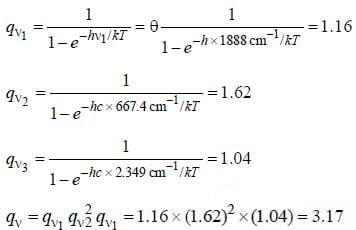QUESTION: 2

### Imagine gaseous Ar at 298k confined to move in a two dimensional plane of area 1.00 cm2. The value of partition funciton is

Solution: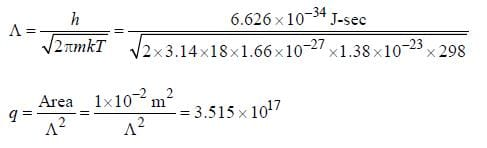QUESTION: 3

### For which of the following molecule is the high temperature expression for the rotational partition function valid if T = 40K is

Solution: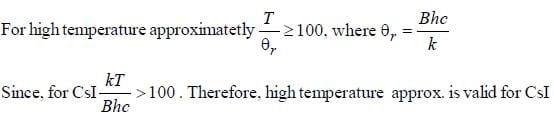QUESTION: 4

The value of rotational partition function for the following species at 298 K is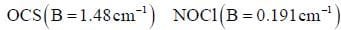Solution:

Since, NOCl is non linear rotor therefore two more rotational constants are required (i.e. A and C)
(Therefore, sufficient information is not provided to solve the question)

QUESTION: 5

Four identical fermion particles are to be distributed in two energy levels E1 and E2. Energy E1 is 5-fold degenerate and E2 is 6-fold degenerate. The energy corresponding to the distribution having maximum number of arrangements is

Solution: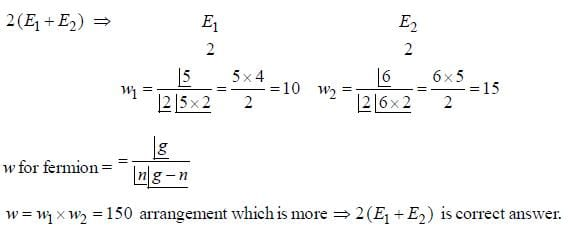QUESTION: 6

The correct form of vibrational partition function is

Solution: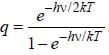QUESTION: 7

The value of temperature at which 10% of the molecule in a system will be in first excited electronic state if this state is 400 kJ/mol above ground state is

Solution: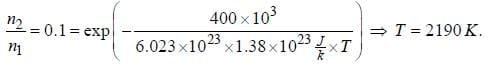*Answer can only contain numeric values
QUESTION: 8

The ground state of Co2+ ion in CoSO4.7H2O may be regarded as 4T3/2. The entropy of the solid at temperature below 1K is derived almost entirely from electron spin. The molar entropy of the solid at these temperature is ________J/mol-K.(Round off to two decimal places).

Solution: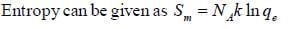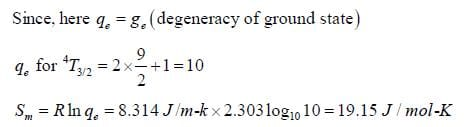*Answer can only contain numeric values
QUESTION: 9

Consider following energy levels with given number of particles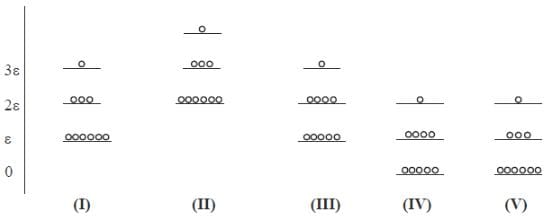If system (III) is undergoing cooling, change in molar entropy of the system is _______J/mole-K. (Round off to one decimal place)

Solution: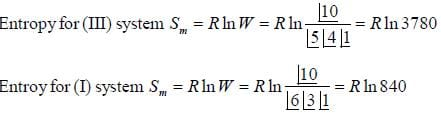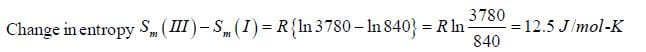*Answer can only contain numeric values
QUESTION: 10

The exact probability distribution of rotational energy levels is the Boltzmann factor of each level Weighted by the degeneracy over the partition function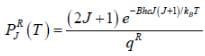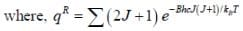The plot of PRJ(T) vs J rotational quantum number for hetronuclear diatomic molecule at 100K is as follows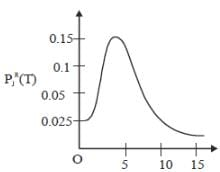If the characteristic rotaional temperature for the molecule is 2.779K. The percentage deviation between exact rotational partition function and the partition function calculated by high-temperature approximation is ____%. (answer should be an integer).

Solution: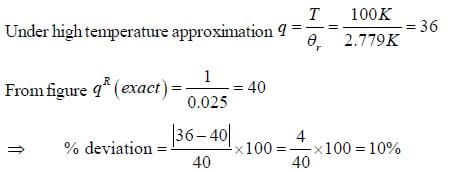QUESTION: 11

The correct form of Sackur Tetrode Equation is

Solution: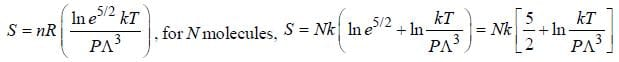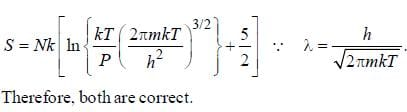QUESTION: 12

The quantity remain constant in Grand Canonical Ensemble

Solution:

is constant is Grand canonical function.

QUESTION: 13

Molar entropy of H-atom at 1000 K and 1 bar is

Solution: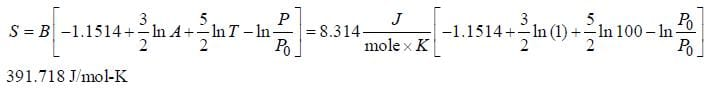*Answer can only contain numeric values
QUESTION: 14

Using Boltzmann porbability distribution expression. The value of possible ways of distributing 7 distingusabe particle among 4 energy levels with energies 0, ε, 2ε and 3ε respectively ____(Total energy of the system remain constant at 3ε)(answer should be an integer).

Solution: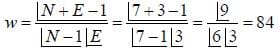*Answer can only contain numeric values
QUESTION: 15

Certain mass of a gas occupy 200mL at 1270C. If the gas is cooled to -730C constant pressure, its new volume _____ mL.(an answer should be an integer).

Solution: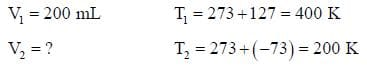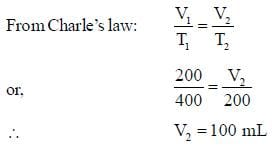QUESTION: 16

In what mass ratio, He and O2 gases should be mixed together to have the same partial pressures is

Solution: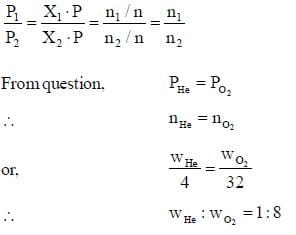*Answer can only contain numeric values
QUESTION: 17

Nickel forms a gaseous compound of the formula Ni(CO)x. The value of x given that under the same conditions of temperature and pressure methane effuses 3.25 times faster than the compound is _____(answer should be an integer). (Given : Ni = 58.7)

Solution: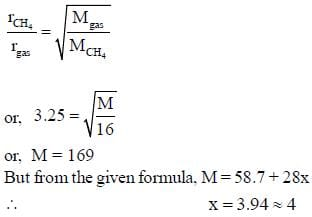*Answer can only contain numeric values
QUESTION: 18

A vessel contains 80g methane at 270C. The total translational kinetic energy of molecules is _____kJ. (Round off to two decimal places)

Solution: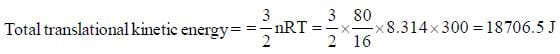*Answer can only contain numeric values
QUESTION: 19

The rate of effusion of an unknown gas, X, at 480K is 1.60 times the rate of effusion of sulphur dioxide gas at 300K. The molecular weight of X is ______ (answer should be an integer).

Solution: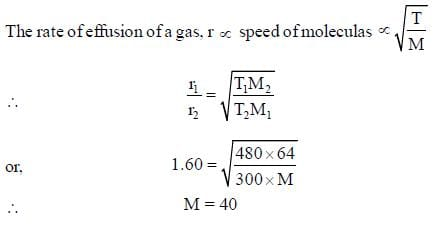*Answer can only contain numeric values
QUESTION: 20

If rotational constant for H2O(g) at 15000K are A = 27.8778cm-1, B = 14.5042cm-1 and C = 9.286cm-1. The value of change in rotational free energy. (Round off to two decimal places).
[Given: Gm - Gm(0) is _____kJmol-1.

Solution: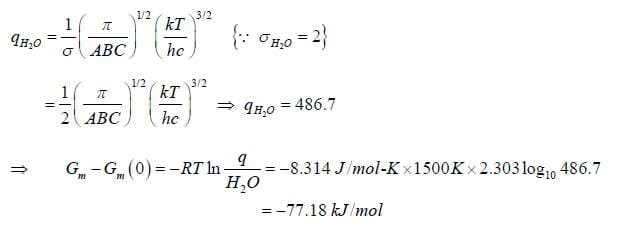Use Code STAYHOME200 and get INR 200 additional OFF Use Coupon Code